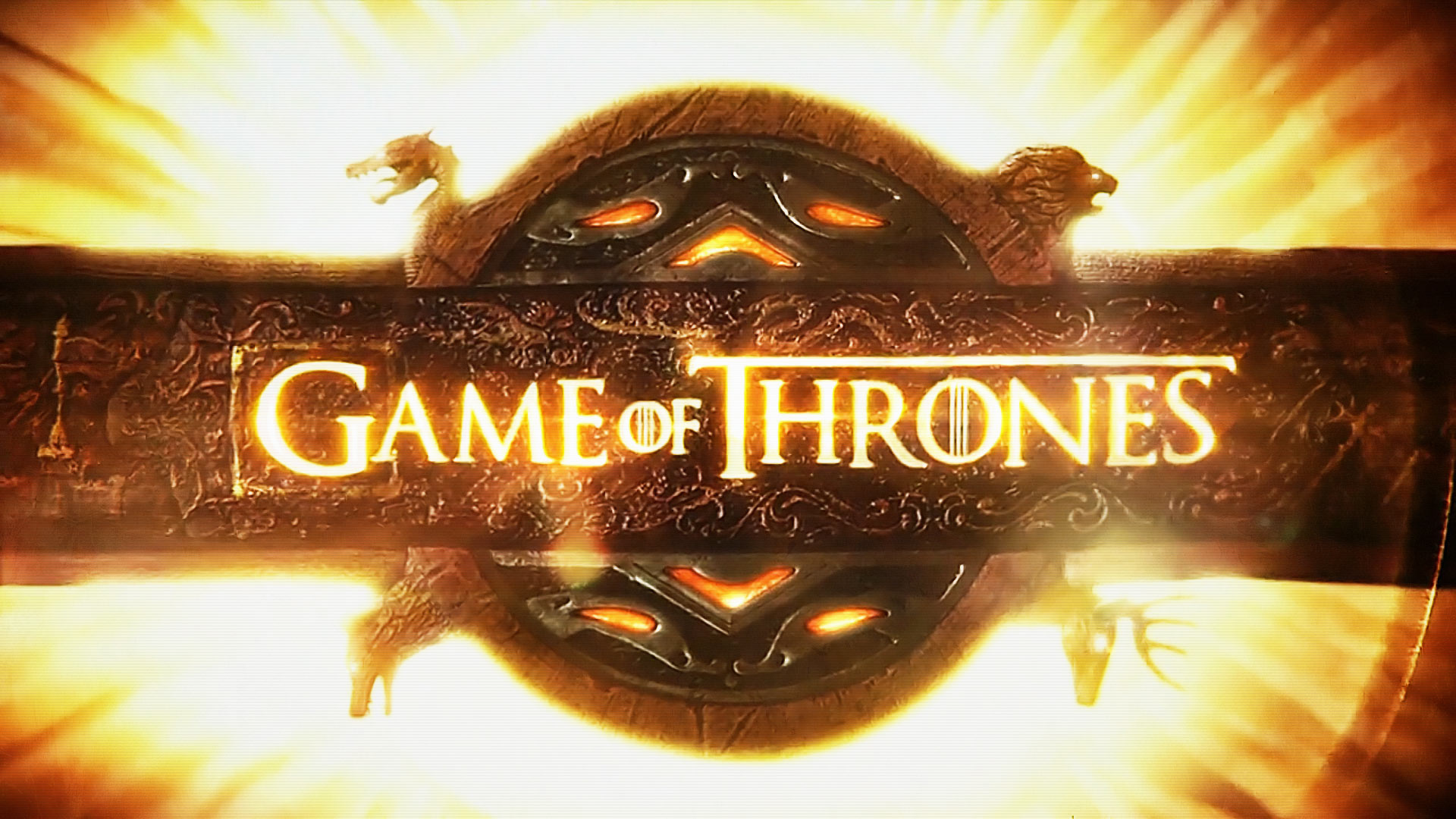There may not be a situation more perilous than being a character on Game of Thrones. Warden of the North, Hand of the King, and apparent protagonist of the entire series? Off with your head before the end of the first season! Last male heir of a royal bloodline? Here, have a pot of molten gold poured on your head! Invited to a wedding? Well, you probably know what happens at weddings in the show.

So what do all these gruesome deaths have to do with statistics? They are data that come from a Poisson distribution.

Data from a Poisson distribution describe the number of times an event occurs in a finite observation space. For example, a Poisson distribution can describe the number of defects in the mechanical system of an airplane, the number of calls to a call center, or in our case it can describe the number of deaths in an episode of Game of Thrones.

## Goodness-of-Fit Test for Poisson

If you're not certain whether your data follow a Poisson distribution, you can use Minitab Statistical Software to perform a goodness-of-fit test. If you don't already use Minitab and you'd like to follow along with this analysis, download the free 30-day trial.

I collected the number of deaths for each episode of Game of Thrones (as of this writing, 57 episodes have aired), and put them in a Minitab worksheet. Then I went to Stat > Basic Statistics > Goodness-of-Fit Test for Poisson to determine whether the data follow a Poisson distribution. You can get the data I used here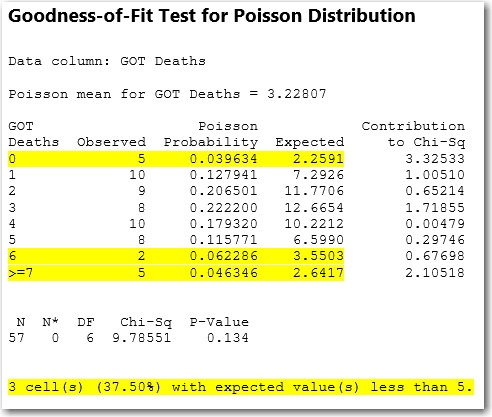Before we interpret the p-value, we see that we have a problem. Three of the categories have an expected value less than 5. If the expected value for any category is less than 5, the results of the test may not be valid. To fix our problem, we can combine categories to achieve the minimum expected count. In fact, we see that Minitab actually already started doing this by combining all episodes with 7 or more deaths.

So we'll just continue by making the highest category 6 or more deaths, and the lowest category 1 or 0 deaths. To do this, I created a new column with the categories 1, 2, 3, 4, 5 and 6. Then I made a frequency column that contained the number of occurrences for each category. For example, the "1" category is a combination of episodes with 0 deaths and 1 death, so there were 15 occurrences. Then I ran the analysis again with the new categories.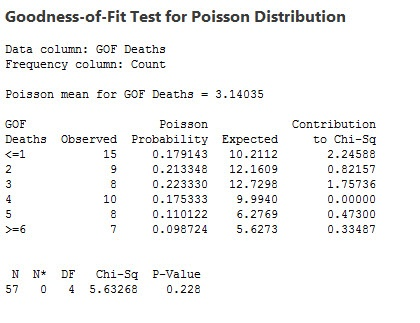Now that all of our categories have expected counts greater than 5, we can examine the p-value. If the p-value is less than the significance level (usually 0.05 works well), you can conclude that the data do not follow a Poisson distribution. But in this case the p-value is 0.228, which is greater than 0.05. Therefore, we cannot conclude that the data do not follow the Poisson distribution, and can continue with analyses that assume the data follow a Poisson distribution.

## Confidence Interval for 1-Sample Poisson Rate

When you have data that come from a Poisson distribution, you can use Stat > Basic Statistics > 1-Sample Poisson Rate to get a rate of occurrence and calculate a range of values that is likely to include the population rate of occurrence. We'll perform the analysis on our data.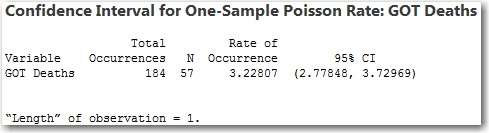The rate of occurrence tells us that on average there are about 3.2 deaths per episode on Game of Thrones. If our 57 episodes were a sample from a much larger population of Game of Thrones episodes, the confidence interval would tell us that we can be 95% confident that the population rate of deaths per episode is between 2.8 and 3.7.

The length of observation lets you specify a value to represent the rate of occurrence in a more useful form. For example, suppose instead of deaths per episode,  you want to determine the number of deaths per season. There are 10 episodes per season. So because an individual episode represents 1/10 of a season, 0.1 is the value we will use for the length of observation.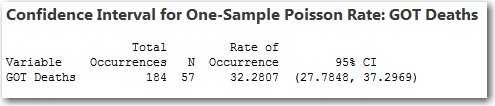With a different length of observation, we see that there are about 32 deaths per season with a confidence interval ranging from 28 to 37.

## Poisson Regression

The last thing we'll do with our Poisson data is perform a regression analysis. In Minitab, go to Stat > Regression > Poisson Regression > Fit Poisson Model to perform a Poisson regression analysis. We'll look at whether we can use the episode number (1 through 10) to predict how many deaths there will be in that episode.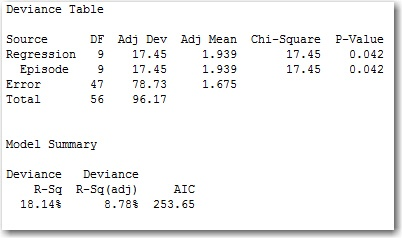The first thing we'll look at is the p-value for the predictor (episode). The p-value is 0.042, which is less than 0.05, so we can conclude that there is a statistically significant association between the episode number and the number of deaths. However, the Deviance R-Squared value is only 18.14%, which means that the episode number explains only 18.14% of the variation in the number of deaths per episode. So while an association exists, it's not very strong. Even so, we can use the coefficients to determine how the episode number affects the number of deaths.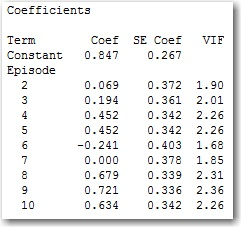The episode number was entered as a categorical variable, so the coefficients show how each episode number affects the number of deaths relative to episode number 1. A positive coefficient indicates that episode number is likely to have more deaths than episode 1. A negative coefficient indicates that episode number is likely to have fewer deaths than episode 1.

We see that the start of each season usually starts slow, as  7 of the 9 episode numbers have positive coefficients. Episodes 8, 9, and 10 have the highest coefficients, meaning relative to the first episode of the season they have the greatest number of deaths. So even though our model won't be great at predicting the exact number of deaths for each episode, it's clear that the show ends each season with a bang.

So, if you're a Game of Thrones viewer you should brace yourself, because death is coming. Or, as they would say in Essos:

Valar morghulis.